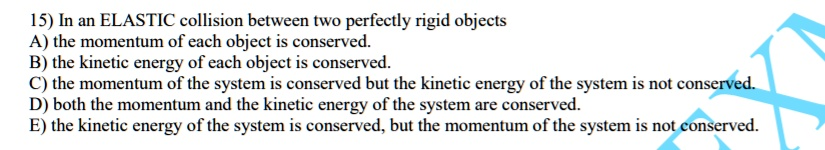5

# 15) In an ELASTIC collision between tWo perfectly rigid objects A) the momentum of each object is conserved. B) the kinetic energy of each object is conserved: the ...

## Question

###### 15) In an ELASTIC collision between tWo perfectly rigid objects A) the momentum of each object is conserved. B) the kinetic energy of each object is conserved: the momentum of the system is conserved but the kinetic energy of the system is not conserved D) both the momentum and the kinetic energy of the system are conserved. E) the kinetic energy of the system is conserved, but the momentum of the system is not conserved.

15) In an ELASTIC collision between tWo perfectly rigid objects A) the momentum of each object is conserved. B) the kinetic energy of each object is conserved: the momentum of the system is conserved but the kinetic energy of the system is not conserved D) both the momentum and the kinetic energy of the system are conserved. E) the kinetic energy of the system is conserved, but the momentum of the system is not conserved.